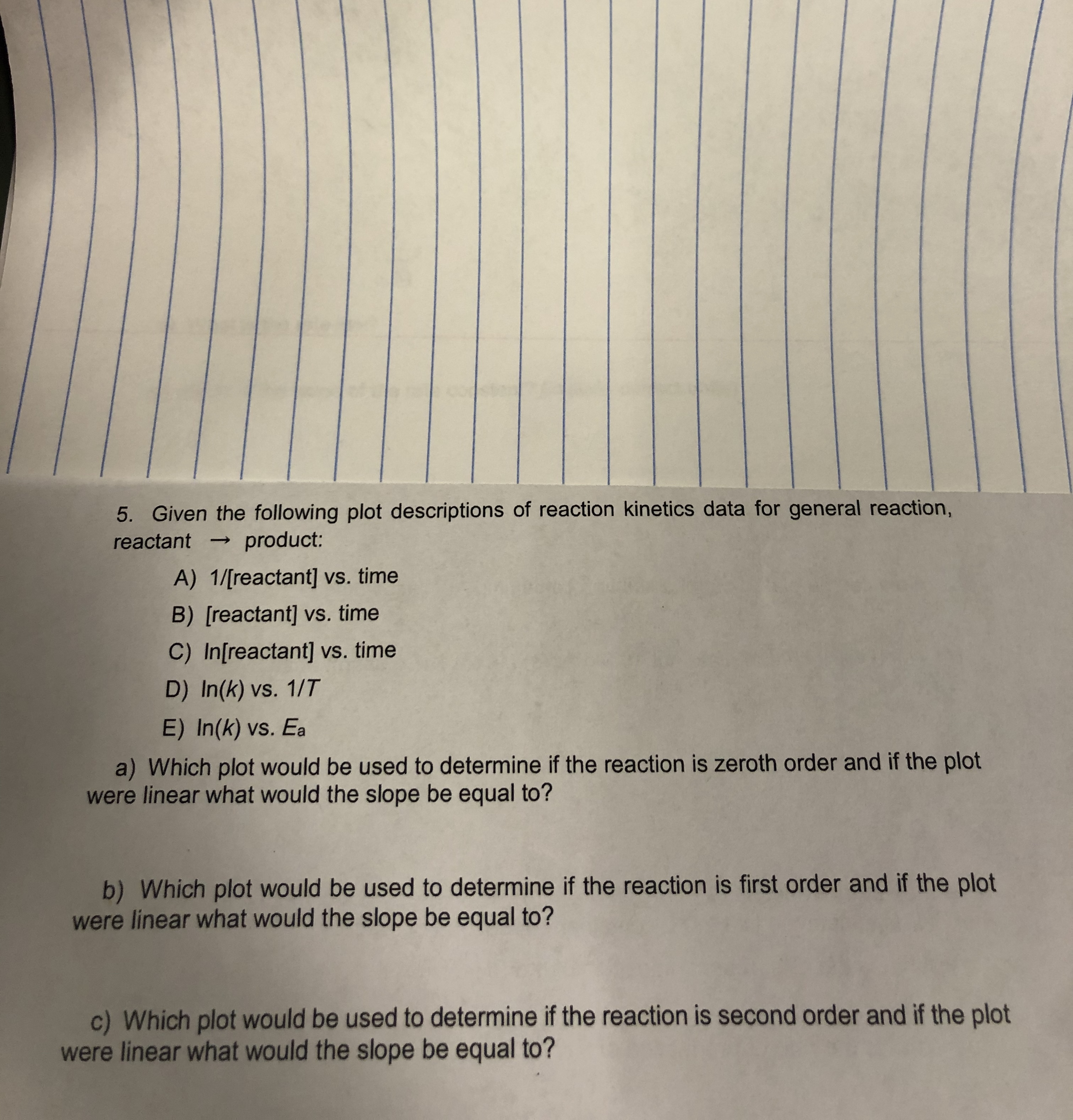# Given the following plot descriptions of reaction kinetics data for general reaction, reactant -> product: a) Which plot would be used to determine if the reaction is zeroth-order and if the plot is linear what would the slope be equal to? b) Which plot would be used to determine if the reaction is first-order and if the plot is linear what would the slope be equal to? c) Which plot would be used to determine if the reaction is second-order and if the plot is linear what would the slope be equal to?